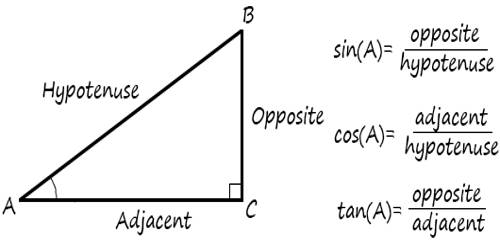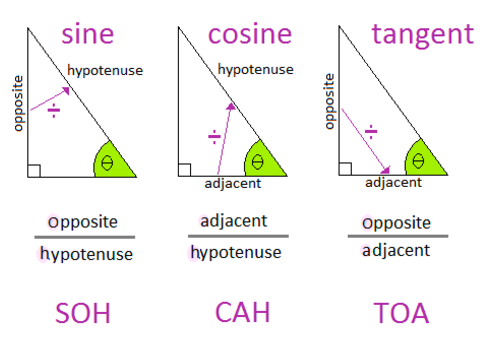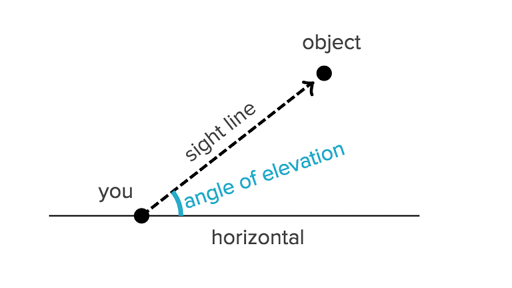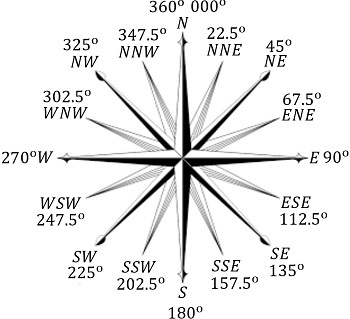# Year 9 Maths Chapter 3 - Right-angled triangles

## “the earth is flat” – our Year 9 maths teacher

You will want to refer to Year 8 Maths Chapter 6 for basic geometry. This section is more advanced, and omits basic concepts.

## Pythag

``````a² + b² = c²
``````

where `a` and `b` is the opposite/adjacent, and `c` is the hypotenuse

Pythagorean triads include 3, 4, 5 (because `3² + 4² = 5²`) and 6, 8, 10 (because `6² + 8² = 10²`).

## Trig ratios

Take a right angled triangle with angle `θ`.• The longest side of the triangle (opposite the right angle) is the hypotenuse.
• The side opposite `θ` is the opposite.
• The side adjacent to `θ` is the adjacent.

There are trig ratios for these sides: sine, cosine, and tangent, which are abbreviated to `sin`, `cos` and `tan` respectively.

In all of the below ratios, the side in the ratio refers to the length of a corresponding side. E.g opposite over adjacent = length of opposite side over length of adjacent side.

1. Sine ratio
``````     opposite
sinθ = ––––––––––
``````
2. Cosine ratio
``````      adjacent
cosθ = ––––––––––––
hypotenuse
``````
3. Tangent ratio
``````     opposite
tanθ = ––––––––––
``````

This can be remembered using the term:

### SOH CAH TOA### Finding sides

1. Find trig ratio
2. With the trig ratio, replace θ with the (acute) angle stated
3. Insert a pronumeral for the unknown side, into the trig ratio
4. Solve### Finding angles

1. Find trig ratio
2. Insert the appropriate sides into their corresponding positions on the trig ratio
3. Use sin⁻¹(your ratio here) to solve for the unknown angle## Angles of elevation and depression

• The angle of elevation is how big the angle between a horizontal and a line of sight is. e.g when you look up, you are forming an angle of elevation
• Similarly, the angle of depression is how big the angle between a horizontal and line of sight is, but instead of being up, it is downoof that meme

## Bearing

Measured clockwise from north## Chapter 3 SummaryCopyright © 2017-2020 aidswidjaja and other contributors. CC BY-SA 4.0 Australia unless otherwise stated.